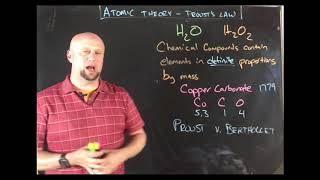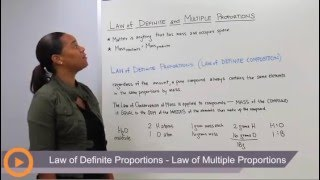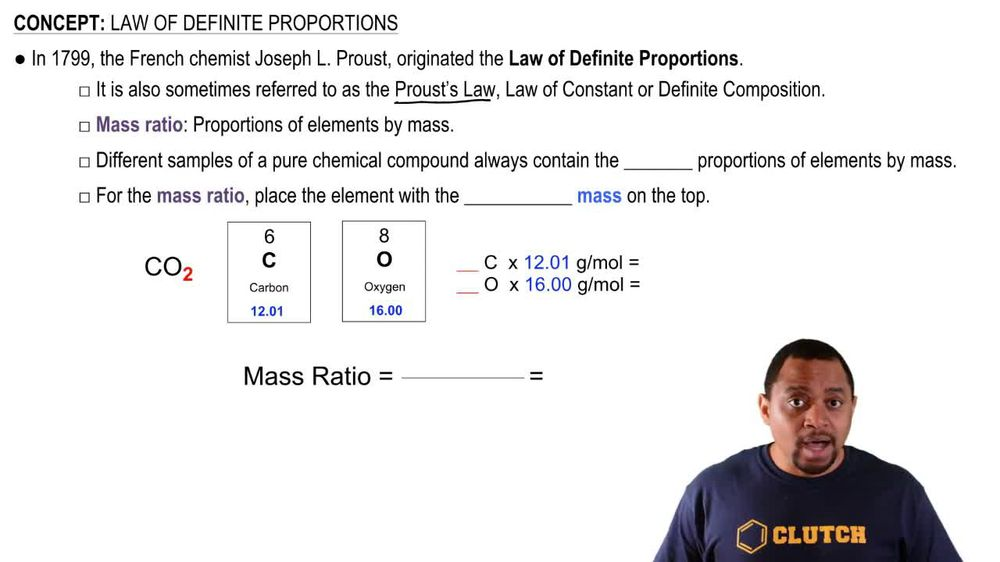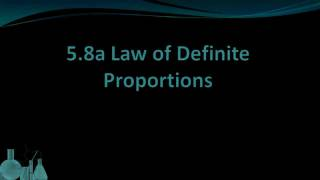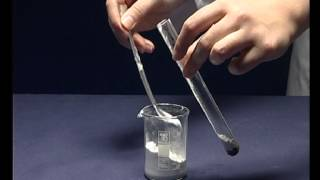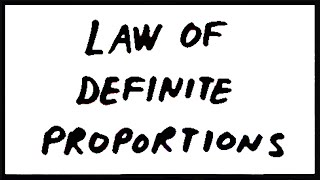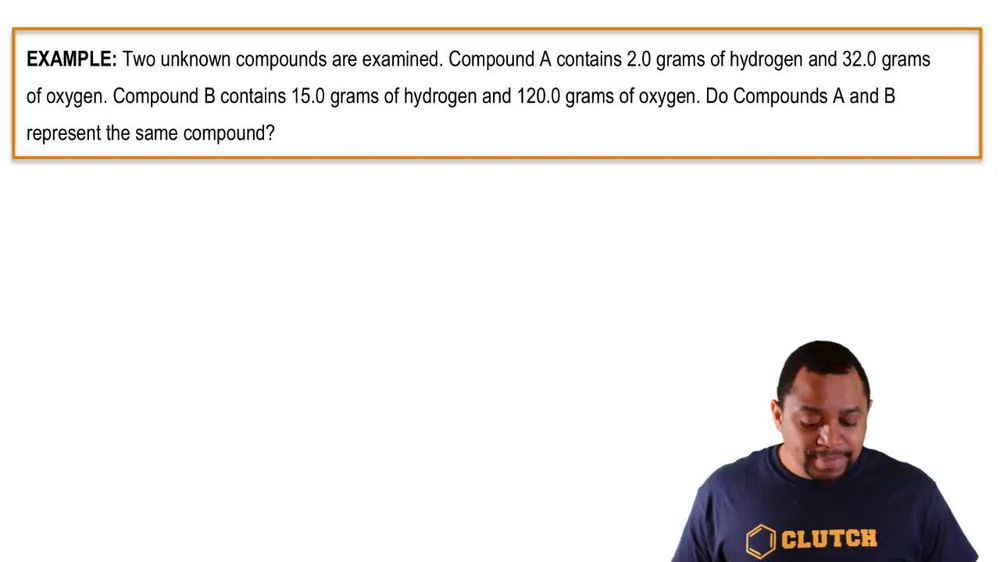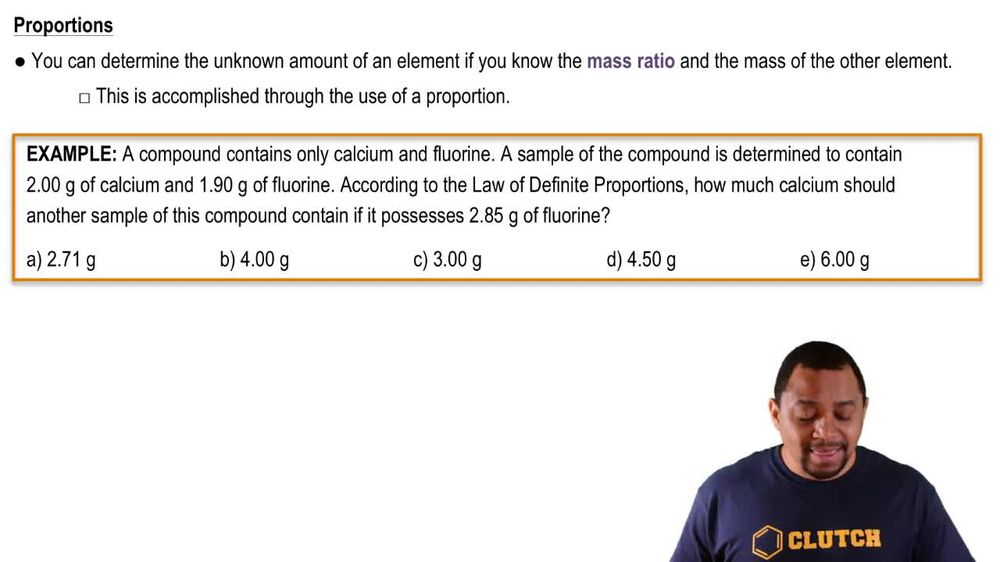Start typing, then use the up and down arrows to select an option from the list.
1. 2. Atoms & Elements2. Law of Definite Proportions
Problem

# Determine the number of each type of atom in each formula. a. Ca(NO2)2

Relevant Solution1m
Play a video:
Hi everyone for this problem. We're being asked to identify the number of atoms for each element in the formula shown below. So let's go ahead and get started. So our first element is magnesium and here we can see that we only have one magnesium. We have a subscript here that isn't shown. And our parentheses around the Cielo three and that to outside of it does not pertain to that magnesium. So let's move on to our next element. We have a chlorine and here it's not written, but we have one chlorine and once we filter it out to include what's outside of the parentheses, we see that that too is going to apply to what's inside. So our one chlorine times two Gives us two chlorine and our last element is oxygen. So we also have three. Oxygen's Multiplied by what's outside of the parentheses is two gives us six. So we have a final answer of one magnesium to chlorine and six oxygen's okay, that is the number of atoms in each element in the formula shown. And that is the end of this problem. I hope this was helpful